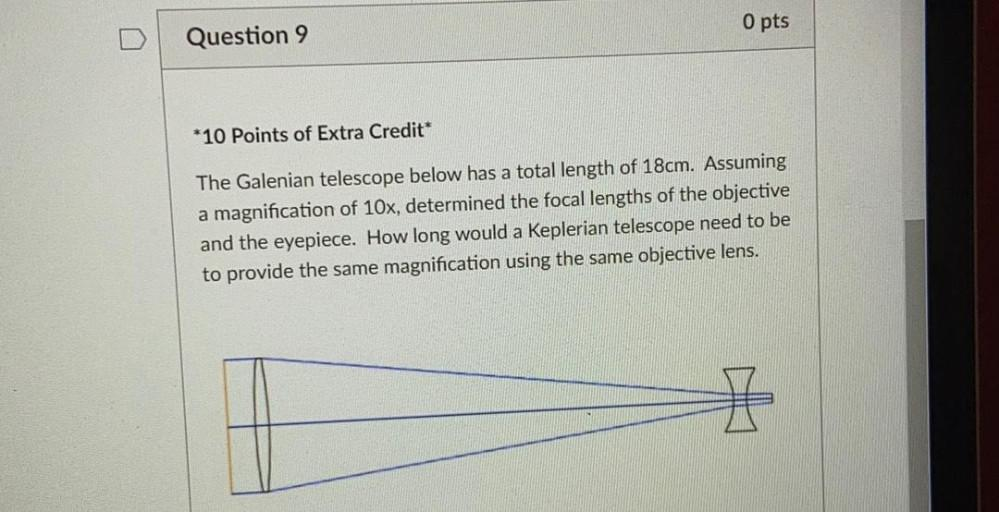Question:

# O pts Question 9 *10 Points of Extra Credit The Galenian telescope below has a total length of 18cm. Assuming a magnification ofO pts Question 9 *10 Points of Extra Credit The Galenian telescope below has a total length of 18cm. Assuming a magnification of 10x, determined the focal lengths of the objective and the eyepiece. How long would a Keplerian telescope need to be to provide the same magnification using the same objective lens.